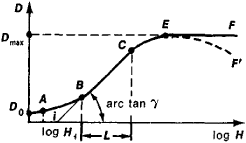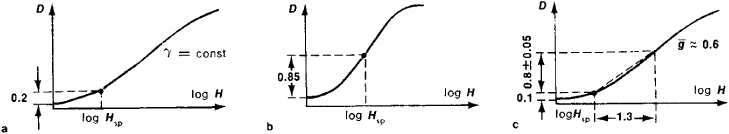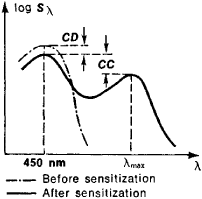# Sensitometry

Also found in: Dictionary, Thesaurus, Medical, Wikipedia.
Related to Sensitometry: sensitometric curve
The following article is from The Great Soviet Encyclopedia (1979). It might be outdated or ideologically biased.

## Sensitometry

the science devoted to the measurement of the photographic properties of light-sensitive materials. Sensitometry arose in the 1840’s after the discovery of photography and for a long period was limited to the determination of the sensitivity of photographic materials to light (hence the name of the field). The scope of sensitometry was considerably broadened after the British scientists F. Hurter and V. Driffield introduced the concept of the characteristic curve in 1890. Many important properties of photographic materials can be evaluated by means of this curve. The present-day practical importance of sensitometry is very great. Sensitometry is the basis for many techniques of quantitative monitoring of individual stages of the production of photographic and motion-picture materials and the mass production of photographic images in, for example, cinematography, printing, and aerial photography. Acceptance testing and testing for arbitration purposes also make use of sensitometry. Sensitometric techniques are widely used in areas of science—such as astronomy and spectroscopy—where the photographic recording of radiation is required.

Sensitometry has three principal branches: densitometry, integral sensitometry, and spectral sensitometry. Densitometry is devoted to the measurement of the optical density of the blackening of developed sensitive layers in order to obtain a quantitative evaluation of the results of the photographic process. The other two branches of sensitometry are concerned with the quantitative expression of the properties of photographic materials on the basis of density measurements. In integral sensitometry, the properties of materials are determined relative to white light or some other light of complex spectral composition. Spectral sensitometry studies the properties—in particular, theFigure 1. Typical characteristic curve of a photographic material: (AB) toe of curve; (BC) straight-line portion of curve; (CE) shoulder of curve; (EF’) region of solarization; (γ) tangent, called gamma, of the angle made by the extension of BC and the log H axis; (L) photographic latitude, which is the projection of BC on the log H axis; (i) inertia point, where the extension of BC intersects the log H axis; (D0) fog density; (A) threshold of blackening; (Dmax) maximum attainable density for the given material. The region of fog is to the left of point A.

spectral sensitivity— of photographic materials as a function of the wavelength of the exposing light and investigates the influence of the nature of these functions on the final photographic effect. According to the types of sensitive materials studied, sensitometry is divided into the sensitometry of black-and-white materials, the sensitometry of color materials, the sensitometry of photographic papers, and the sensitometry of materials for recording ionizing radiation. Instruments used in sensitometry include densitometers, microphotometers, sensitometers, spectrosensitometers, and resolution testers.

The aggregate of the interrelated methods of testing the photographic properties of light-sensitive materials constitutes a sensitometric system. The Soviet sensitometric system is based on GOST’s (All-Union State Standards) 2817–50, 2818–45, 2919–45, and 10691–63 for black-and-white materials and GOST 9160–59 for color materials. The difference between the Soviet system and other national systems—such as the American ASA and German DIN systems—is that the Soviet system provides more complete information and involves general sensitometric testing, spectrosensitometric testing, and determination of the resolving power of the photographic materials.

The characteristic curve of a photographic material (Figure 1) expresses the dependence of the optical density D of the blackening that results from development on the logarithm of the exposure log H. The tangent γ of the angle made by the straight-line portion of the curve and the log H axis is called gamma. It characterizes the ability of the material to convey the difference in brightness of different parts of the scene being photographed. The logarithm of the ratio of exposures between which the characteristic curve is a straight line is called the photographic, or exposure, latitude L; in Figure 1, L = log HC/HB. In practical photography, the greater the value of the L, the better.

A very important sensitometric quantity is the speed, or sensitivity, S of a photographic material. This quantity is determined by the characteristic curve and is used in comparing materials and selecting the optimal exposure conditions in still photography, motion-picture filming, and the printing of positives. The speed is inversely proportional to the exposure corresponding to a certain point, called the speed point, on the characteristic curve: S = k/Hsp, where k is a constant. In the case of general-purpose black-and-white materials and black-and-white motion-picture film (for white light of a given spectral composition), the Soviet sensitometric system uses a speed criterion based on a diffuse density of 0.2 above the fog density D0; for black-and-white film used in aerial photography, the speed point is located at 0.85 above D0. Thus,

S0.2 = 1/HD = 0.2+D0

and

S0.85 =10/HD = 0.85+D0

These two cases are illustrated in Figure 2. H is expressed in lux-seconds. In the ASA system,

S0.1 = 0.8/HD = 0.1+D0

and in the DIN system,

S = 101og 1/HD=0.1 + D0Figure 2. Various criteria for the determination of the speed of photographic materials on the basis of characteristic curves: (a) GOST system, wherein the speed criterion is based on a density of 0.2 above the fog density; (b) supplementary GOST criterion, which is based on a density of 0.85 above the fog density; (c) DIN and ASA systems, wherein the speed criterion is based on a density of 0.1 above the fog density (in addition, it is required that the density increase by a given factor g over the portion of the curve used—the length of the projection of this portion on the log H axis must be 1.3)

In other words, in both the ASA and DIN systems, speed is based on a diffuse optical density of 0.1 above fog density.

The average accuracy of the determination of speed is ±10 percent; for gamma, the average accuracy is ±4 percent. Fog density is determined with an accuracy of ±0.02 density units.

Since S, γ, and D0 depend on the development time tdev, general sensitometric testing involves the plotting of the curves S = f1 (tdev). γ = f2 (tdev). and D0 = f3 (tdev), which are sometimes called development kinetics curves. These curves are used to determine the speed number, an important parameter for comparing photographic materials and selecting the correct exposure conditions in practical photography. The speed number is the speed when gamma is equal to what is called the recommended gamma γrec. For general-purpose, or amateur, photographic films, γrec = 0.8; γrec = 0.65 for negative motion-picture movie films, and γrec = 1.8 for positive motion-picture films. The speed number S0.2 of present-day negative materials ranges from ∼20 to 350–400 GOST units, and S0.85 of films for aerial photography varies from 130 to 2,000 GOST units. The following relations hold between the speeds of photographic materials of average contrast (γ = 0.8–1) in the Soviet system and the principal non-Soviet systems: SG0ST = 0.65 antilog 0.1SDIN and SGOST = 0.8SASA. Thus, a speed of 32 GOST units is equivalent to 17° DIN and to 40 ASA. Sensitometric testing also involves the plotting of reciprocity curves for constant density (seeRECIPROCITY LAW FAILURE)

In color materials, the color image is produced by three dyes in three emulsions coated one above another. For this reason, the principal relationships—such as characteristic curves, reciprocity curves, and development kinetics curves—are each expressed by sets of three curves, and the numerical sensitometric parameters are each given by three quantities. These quantities correspond to the three layers (analytical evaluation), or, in some cases, to the types of basic receptors of a color-sensitive detector of radiation coming as an integral whole from a color image (integral evaluation). For negative sensitive layers, such receptors are layers of positive material (a print is produced on the positive layers from a negative image). For the evaluation of positive material, the receptors are the color-sensitive elements of an eye examining the image.

In testing black-and-white photographic materials, the techniques of spectral sensitometry are used to find the dependence of the radiometric spectral sensitivity Sλ, and the monochromatic gamma γλ on the wavelength λ of the exposing monochromatic light. Sλ is usually defined as

Sλ = 1/HD = 1.0+D0

and is expressed in terms of radiometric quantities—for example. cm2/erg. Spectral sensitivity curves log Sλ = f(λ) are used to determine the degree of optical sensitization (Figure 3) and the limiting wavelength λlim beyond which the material is essentially insensitive to radiation. The principal instrument used in spectral sensitometry is the spectrosensitometer.

Resolution testing determines the resolving power of a photographic material—that is, the material’s ability to reproduce fine detail—and a number of other related characteristics. In such testing, a resolution curve vlim = f(log H) is obtained, where vlim is the maximum number of lines per millimeter that can be visually separated when the exposure is H. Resolution testing also involves the determination of the modulation transmission function for the blue and red spectral regions.

A separate branch of sensitometry studies the photographic properties of positive printing papers. The maximum density ofFigure 3. Spectral sensitivity curve of a sensitized photographic material: (CC) degree of sensitization, (CD) degree of desensitization (the decrease in the maximum value of log Sλ in the region of the material’s intrinsic sensitivity), (λmax) wavelength corresponding to the maximum additional sensitivity resulting from sensitization

blackening and the useful exposure range (a quantity analogous to the photographic latitude) of photographic papers are considerably less than for positive materials on a transparent base. For this reason, the results of sensitometric testing for papers are expressed in ways different from those used for films and plates. The densitometry of photographic papers is also substantially different because the bases of papers are opaque and density is measured by means of reflected rather than transmitted light.

### REFERENCES

Gorokhovskii, Iu. N., and T. M. Levenberg. Obshchaia sensitometriia: Teoriia i praktika. Moscow, 1963.
Gorokhovskii, Iu. N., and V. P. Baranova. Svoistva cherno-belykh fotograficheskikhplenok. Moscow, 1970.
Gorokhovskii. Iu. N. Spektral’nye issledovaniia folograficheskogo protsessa. Moscow. 1960.
Mees, C., and T. James. Teoriia fotograficheskogo protsessa. Leningrad. 1973. (Translated from English.)

L. N. KAPORSKII